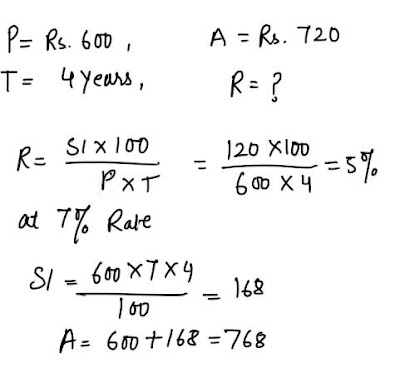# Simple Interest and Compound Interest - 12 Repeated Questions in Exams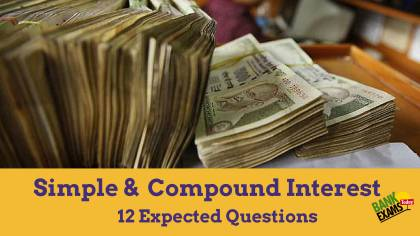#### Ques 1.

A sum was invested at simple interest at a certain interest for 2 years. It would have fetched Rs. 60. more had it been invested at 2% higher rate. What was the sum
(a) Rs 1500
(b) Rs 1300
(c) Rs 2500
(d) Rs 1000
Ans 1. Since SI si directly proportional to Rate of interest 2 % higher rate fetched Rs. 60 more
It means  this sum will give SI of Rs. 60
If invested at 2% rate for  2 years#### Ques 2.

The difference between simple and compound interest on a sum of money at 5% per annum is Rs .25 .What is the sum ?'
(a) Rs 5000
(b) Rs 10,000
(c) Rs. 4000
(d) Data insufficient
Ans 2.  It cannot be determined

#### Ques 3.

Two equal sums were borrowed at 8% simple interest per annum for 2 years and 3 years respectively.The difference on the interest was Rs 56.The sum borrowed were
(a) Rs . 690
(b) Rs . 700
(c) Rs. 740
(d) Rs 780
Ans 3.#### Ques 4.

If the difference between the simple interest and compound interest on some prinicpal amount at 20% per annum for 3 years in Rs 48,then the principle amount must be
(a) Rs. 550
(b) Rs. 500
(c) Rs. 375
(d) Rs. 400
Ans 4.#### Ques 5.

Raju lent Rs. 400 to Ajay for 2 years, and Rs. 100 to Manoj for 4 Years and received together form both Rs. 60 as interest .Find the rate of interest, if simple interest is being calculated.
(a) 5 %
(b) 6 %
(c) 8%
(d) 9 %
Ans 5.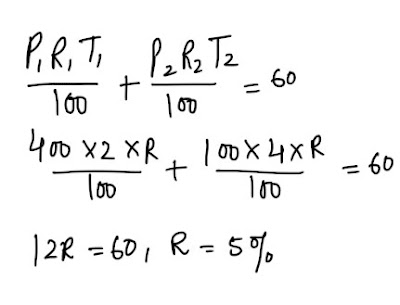#### Ques 6.

In what time will Rs 8000 amount to 40,000 at 4% per annum ? ( simple interest being reckoned )
(a) 100 years
(b) 50 years
(c) 110 years
(d) 160 years
Ans 6.#### Ques 7.

A sum of money becomes 4 times at simple interest i 10 years.What is the rate of interest?
(a) 10%
(b) 20 %
(c) 30%
(d) 40%
Ans 7.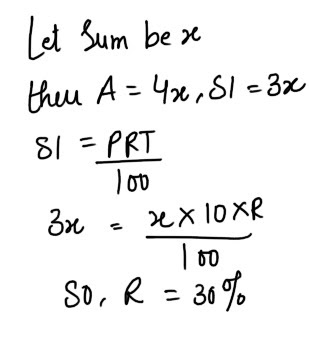#### Ques 8.

A sum of money placed at compound interest doubles itself in 3 years .In how many years will it amount to 8 times itself?
(a) 9 years
(b) 8 years
(c) 27 years
(d) 7 years
Ans 8.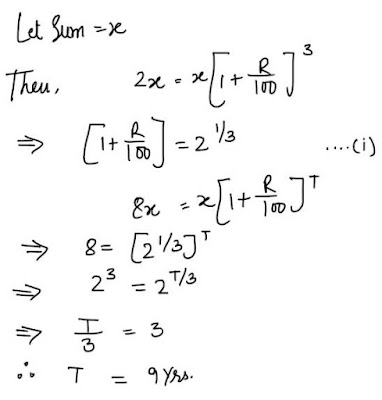#### Ques 9.

Divide Rs 6000 into two parts so that simple interest on the first part for 2 years at 6 % p.a. may be equal to the simple interest on the second part for 3 years at 8% p.a.
(a) Rs. 4000, Rs 2000
(b) Rs. 5000. Rs 1000
(c) Rs. 3000, Rs 3000
(d) None of these
Ans 9.#### Ques 10

A sum of money becomes 7/4 of itself in 6 years at a certain rate of simple interest. Find the rate of interest.
(a) 12%
(b) 12.5%
(c) 8%
(d) 14%
Ans 10.#### Ques 11.

Sanjay borrowed Rs.900 at 4 % p.a. and Rs 1100 at 5% p.a. for the same duration.He had to pay Rs. 364 in all as interest .What is the time period in years?
(a) 5 Years
(b) 3 Years
(c) 2 years
(d) 4 years
Ans 11.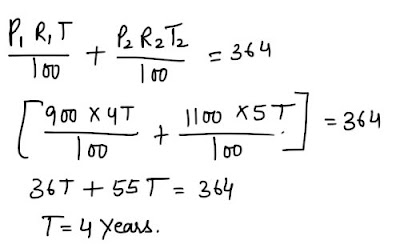#### Ques 12.

A sum of Rs. 600 amounts to Rs. 720 in 4 years at simple Interest. What will it amount to if the rate of interest in increased by 2 % ?
(a) Rs. 648
(b) Rs. 768
(c) Rs. 726
(d) Rs. 792

Ans 12.